# Video Stabilization Using Point Feature Matching

This example shows how to stabilize a video that was captured from a jittery platform. One way to stabilize a video is to track a salient feature in the image and use this as an anchor point to cancel out all perturbations relative to it. This procedure, however, must be bootstrapped with knowledge of where such a salient feature lies in the first video frame. In this example, we explore a method of video stabilization that works without any such a priori knowledge. It instead automatically searches for the "background plane" in a video sequence, and uses its observed distortion to correct for camera motion.

This stabilization algorithm involves two steps. First, we determine the affine image transformations between all neighboring frames of a video sequence using the `estgeotform2d` function applied to point correspondences between two images. Second, we warp the video frames to achieve a stabilized video. We will use the Computer Vision Toolbox™, both for the algorithm and for display.

### Step 1. Read Frames from a Movie File

Here we read in the first two frames of a video sequence. We read them as intensity images since color is not necessary for the stabilization algorithm, and because using grayscale images improves speed. Below we show both frames side by side, and we produce a red-cyan color composite to illustrate the pixel-wise difference between them. There is obviously a large vertical and horizontal offset between the two frames.

```filename = 'shaky_car.avi'; hVideoSrc = VideoReader(filename); imgA = im2gray(im2single(readFrame(hVideoSrc))); % Read first frame into imgA imgB = im2gray(im2single(readFrame(hVideoSrc))); % Read second frame into imgB figure; montage({imgA,imgB}); title(['Frame A',repmat(' ',[1 50]),'Frame B']);```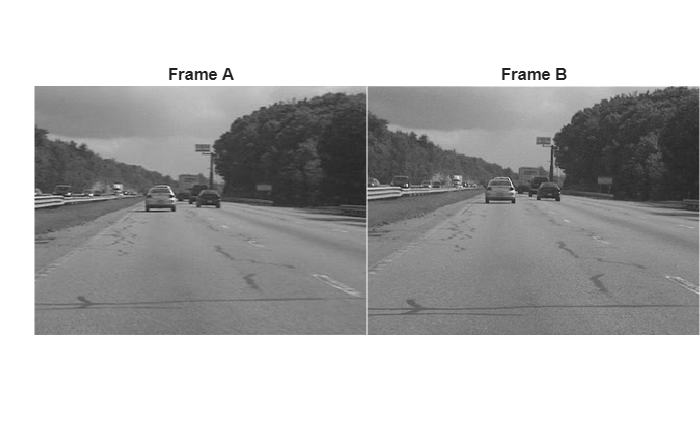```figure imshowpair(imgA,imgB,ColorChannels='red-cyan'); title('Color composite (frame A = red, frame B = cyan)');```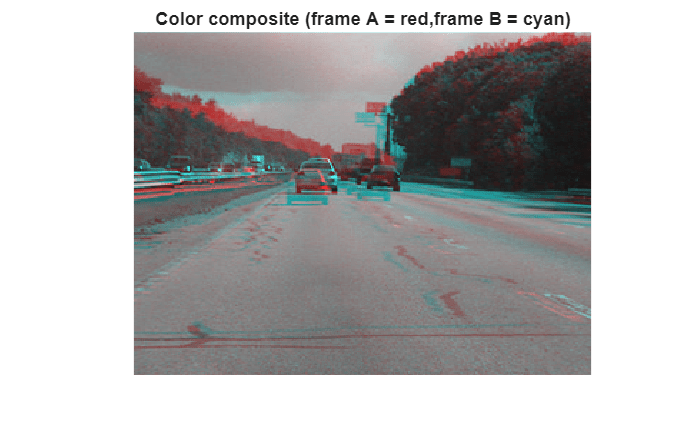### Step 2. Collect Salient Points from Each Frame

Our goal is to determine a transformation that will correct for the distortion between the two frames. We can use the `estgeotform2d` function for this, which will return an affine transformation. As input we must provide this function with a set of point correspondences between the two frames. To generate these correspondences, we first collect points of interest from both frames, then select likely correspondences between them.

In this step we produce these candidate points for each frame. To have the best chance that these points will have corresponding points in the other frame, we want points around salient image features such as corners. For this we use the `detectFASTFeatures` function, which implements one of the fastest corner detection algorithms.

The detected points from both frames are shown in the figure below. Observe how many of them cover the same image features, such as points along the tree line, the corners of the large road sign, and the corners of the cars.

```ptThresh = 0.1; pointsA = detectFASTFeatures(imgA,MinContrast=ptThresh); pointsB = detectFASTFeatures(imgB,MinContrast=ptThresh); % Display corners found in images A and B. figure imshow(imgA) hold on plot(pointsA) title('Corners in A')``````figure imshow(imgB) hold on plot(pointsB) title('Corners in B')```### Step 3. Select Correspondences Between Points

Next we pick correspondences between the points derived above. For each point, we extract a Fast Retina Keypoint (FREAK) descriptor centered around it. The matching cost we use between points is the Hamming distance since FREAK descriptors are binary. Points in frame A and frame B are matched putatively. Note that there is no uniqueness constraint, so points from frame B can correspond to multiple points in frame A.

```% Extract FREAK descriptors for the corners [featuresA,pointsA] = extractFeatures(imgA,pointsA); [featuresB,pointsB] = extractFeatures(imgB,pointsB);```

Match features which were found in the current and the previous frames. Since the FREAK descriptors are binary, the `matchFeatures` function uses the Hamming distance to find the corresponding points.

```indexPairs = matchFeatures(featuresA,featuresB); pointsA = pointsA(indexPairs(:,1),:); pointsB = pointsB(indexPairs(:,2),:);```

The image below shows the same color composite given above, but added are the points from frame A in red, and the points from frame B in green. Yellow lines are drawn between points to show the correspondences selected by the above procedure. Many of these correspondences are correct, but there is also a significant number of outliers.

```figure showMatchedFeatures(imgA,imgB,pointsA,pointsB) legend('A','B')```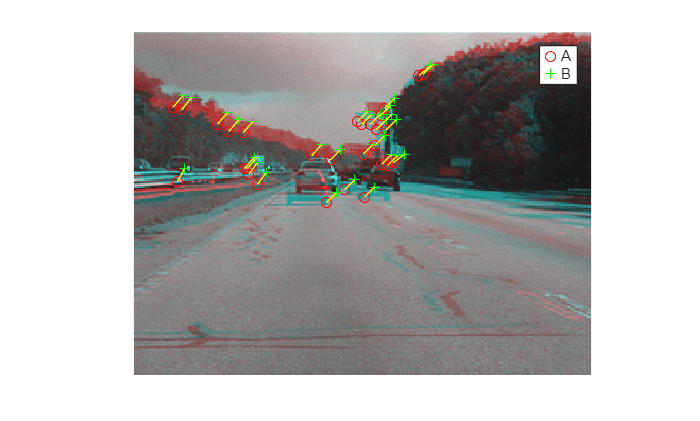### Step 4. Estimating Transformations from Noisy Correspondences

Many of the point correspondences obtained in the previous step are incorrect. But we can still derive a robust estimate of the geometric transformation between the two images using the M-estimator SAmple Consensus (MSAC) algorithm, which is a variant of the RANSAC algorithm. The MSAC algorithm is implemented in the `estgeotform2d` function. This function, when given a set of point correspondences, will search for the valid inlier correspondences. From these it will then derive the affine transformation that makes the inliers from the first set of points match most closely with the inliers from the second set. This affine transformation will be a 3-by-3 matrix of the form:

```[a_1 a_2 t_x; a_3 a_4 t_y; 0 0 1] ```

The parameters $a$ define scale, rotation, and shearing effects of the transformation, while the parameters $t$ are translation parameters. This transformation can be used to warp the images such that their corresponding features will be moved to the same image location.

A limitation of the affine transformation is that it can only alter the imaging plane. Thus it is ill-suited to finding the general distortion between two frames taken of a 3-D scene, such as with this video taken from a moving car. But it does work under certain conditions that we shall describe shortly.

```[tform,inlierIdx] = estgeotform2d(pointsB,pointsA,'affine'); pointsBm = pointsB(inlierIdx,:); pointsAm = pointsA(inlierIdx,:); imgBp = imwarp(imgB,tform,OutputView=imref2d(size(imgB))); pointsBmp = transformPointsForward(tform,pointsBm.Location);```

Below is a color composite showing frame A overlaid with the reprojected frame B, along with the reprojected point correspondences. The results are excellent, with the inlier correspondences nearly exactly coincident. The cores of the images are both well aligned, such that the red-cyan color composite becomes almost purely black-and-white in that region.

Note how the inlier correspondences are all in the background of the image, not in the foreground, which itself is not aligned. This is because the background features are distant enough that they behave as if they were on an infinitely distant plane. Thus, even though the affine transformation is limited to altering only the imaging plane, here that is sufficient to align the background planes of both images. Furthermore, if we assume that the background plane has not moved or changed significantly between frames, then this transformation is actually capturing the camera motion. Therefore correcting for this will stabilize the video. This condition will hold as long as the motion of the camera between frames is small enough, or, conversely, if the video frame rate is high enough.

```figure showMatchedFeatures(imgA,imgBp,pointsAm,pointsBmp) legend('A','B')```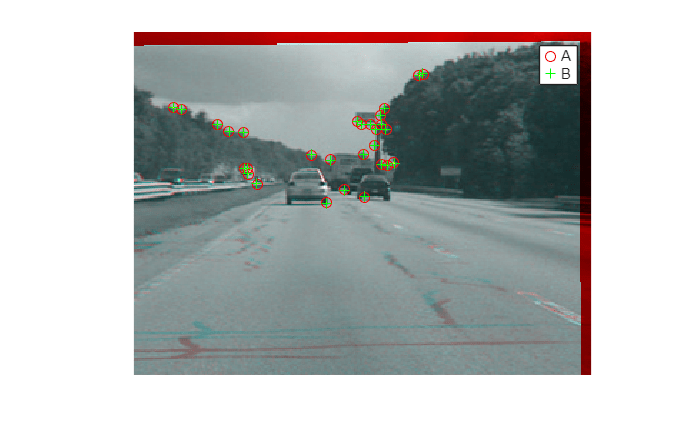### Step 5. Transformation Approximation and Smoothing

Given a set of video frames ${T}_{i},\phantom{\rule{1em}{0ex}}i=0,1,2\dots$, we can now use the above procedure to estimate the distortion between all frames ${T}_{i}$ and ${T}_{i+1}$ as affine transformations, ${H}_{i}$. Thus the cumulative distortion of a frame $i$ relative to the first frame will be the product of all the preceding inter-frame transformations, or

`${H}_{cumulative,i}={H}_{i}\prod _{j=0}^{i-1}$`

We could use all the six parameters of the affine transformation above, but, for numerical simplicity and stability, we choose to re-fit the matrix as a simpler scale-rotation-translation transformation. This has only four free parameters compared to the full affine transformation's six: one scale factor, one angle, and two translations. This new transformation matrix is of the form:

```[s*cos(ang) s*sin(ang) t_x; s*-sin(ang) s*cos(ang) t_y; 0 0 1] ```

We show this conversion procedure below by fitting the above-obtained transformation $H$ with a scale-rotation-translation equivalent, ${H}_{sRt}$. To show that the error of converting the transformation is minimal, we reproject frame B with both transformations and show the two images below as a red-cyan color composite. As the image appears black and white, obviously the pixel-wise difference between the different reprojections is negligible.

```% Extract scale and rotation part sub-matrix tformAffine = tform.A; R = tformAffine(1:2,1:2); % Compute theta from mean of two possible arctangents theta = mean([atan2(-R(3),R(1)) atan2(R(2),R(4))]); % Compute scale from mean of two stable mean calculations scale = mean(R([1 4])/cos(theta)); % Convert theta to degrees thetad = rad2deg(theta); % Translation remains the same translation = tformAffine(1:2,3); % Reconstitute new s-R-t transformation and store it in a simtform2d object tformSRT = simtform2d(scale,thetad,translation); imgBold = imwarp(imgB,tform,OutputView=imref2d(size(imgB))); imgBsRt = imwarp(imgB,tformSRT,OutputView=imref2d(size(imgB))); figure(2) clf imshowpair(imgBold,imgBsRt,'ColorChannels','red-cyan'), axis image; title('Color composite of affine and similarity outputs');```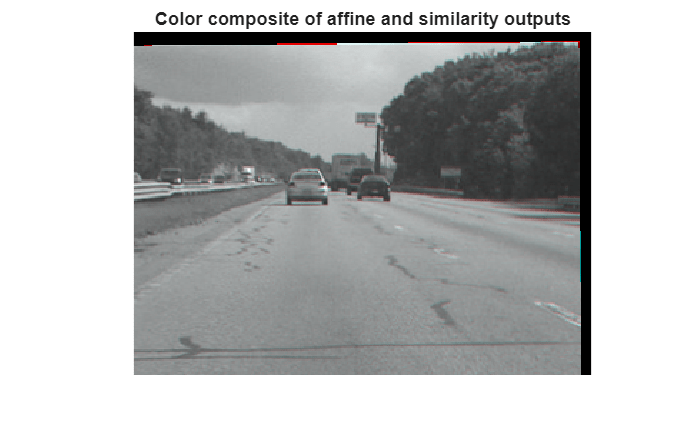### Step 6. Run on the Full Video

Now we apply the above steps to smooth a video sequence. For readability, the above procedure of estimating the transformation between two images has been placed in the MATLAB® function `cvexEstStabilizationTform``.` The function `cvexTformToSRT`` `also converts a general affine transformation into a similarity (scale-rotation-translation) transformation.

At each step we calculate the transformation $H$ between the present frames. We fit this as an s-R-t transform, ${H}_{sRt}$. Then we combine this into the cumulative transformation, ${H}_{cumulative}$, which describes all camera motion since the first frame. The last two frames of the smoothed video are shown in a Video Player as a red-cyan composite.

With this code, you can also take out the early exit condition to make the loop process the entire video.

```% Reset the video source to the beginning of the file read(hVideoSrc,1); hVPlayer = vision.VideoPlayer; % Create video viewer % Process all frames in the video movMean = im2gray(im2single(readFrame(hVideoSrc))); imgB = movMean; imgBp = imgB; correctedMean = imgBp; ii = 2; cumulativeTform = simtform2d; while hasFrame(hVideoSrc) && ii < 10 % Read in new frame imgA = imgB; % z^-1 imgAp = imgBp; % z^-1 imgB = im2gray(im2single(readFrame(hVideoSrc))); movMean = movMean + imgB; % Estimate transformation from frame A to frame B, and fit as an s-R-t tformAffine = cvexEstStabilizationTform(imgA,imgB); sRtTform = cvexTformToSRT(tformAffine); cumulativeTform = simtform2d(cumulativeTform.A * sRtTform.A); imgBp = imwarp(imgB,cumulativeTform,OutputView=imref2d(size(imgB))); % Display as color composite with last corrected frame step(hVPlayer,imfuse(imgAp,imgBp,ColorChannels='red-cyan')); correctedMean = correctedMean + imgBp; ii = ii+1; end correctedMean = correctedMean/(ii-2); movMean = movMean/(ii-2); % Here you call the release method on the objects to close any open files % and release memory. release(hVPlayer);```During computation, we computed the mean of the raw video frames and of the corrected frames. These mean values are shown side-by-side below. The left image shows the mean of the raw input frames, proving that there was a great deal of distortion in the original video. The mean of the corrected frames on the right, however, shows the image core with almost no distortion. While foreground details have been blurred (as a necessary result of the car's forward motion), this shows the efficacy of the stabilization algorithm.

```figure montage({movMean,correctedMean}) title(['Raw input mean',repmat(' ',[1 30]),'Corrected sequence mean'])```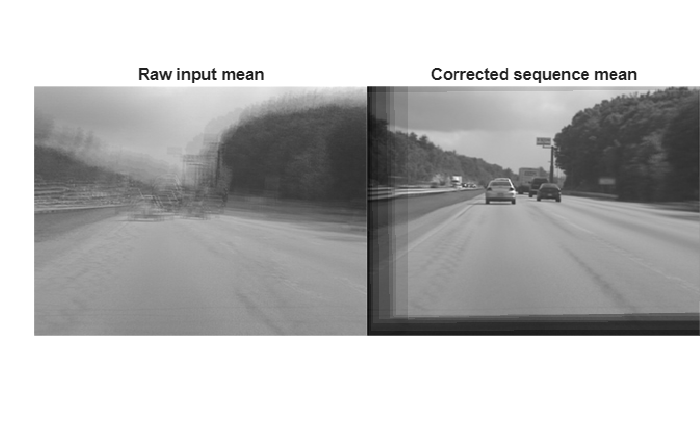### References

 Tordoff, B; Murray, DW. "Guided sampling and consensus for motion estimation." European Conference n Computer Vision, 2002.

 Lee, KY; Chuang, YY; Chen, BY; Ouhyoung, M. "Video Stabilization using Robust Feature Trajectories." National Taiwan University, 2009.

 Litvin, A; Konrad, J; Karl, WC. "Probabilistic video stabilization using Kalman filtering and mosaicking." IS&T/SPIE Symposium on Electronic Imaging, Image and Video Communications and Proc., 2003.

 Matsushita, Y; Ofek, E; Tang, X; Shum, HY. "Full-frame Video Stabilization." Microsoft® Research Asia. CVPR 2005.